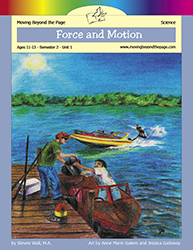# Force and Motion Age 11-13: Concept 2 - Semester 2: Unit 1Why do things move in certain ways? And how can you make them move faster or slower? These are questions that engineers wonder about when they design cars and spaceships and roller coasters. The key to understanding them lies in three laws of motion discovered over 300 years ago.

In this unit, you will learn about different types of forces and how they cause objects to move in certain ways. You'll also learn about how different machines make use of forces and motion to help people get work done.

#### Other Items You May Need

The Age 11-13 semester 2 science units require materials from the Semester 2 Science Kit.
\$110.00 #817 Age 11-13 - Semester 2 - Science Kit

## Prerequisites

• Able to write multiple paragraphs on a topic
• Familiar with the five-paragraph essay
• Usually used by children in the seventh grade.

• Lesson 1: Force
• Lesson 2: Newton's Laws of Motion (2 Days)
• Lesson 3: Graphing Motion
• Lesson 4: Speed, Velocity, and Acceleration (2 Days)
• Lesson 5: Centripetal Force and Terminal Velocity
• Lesson 6: Work (3 Days)
• Lesson 7: Newton in the Milky Way
• Final Project: Demonstrating Newton's Laws (2 Days)

## Summary of Skills

Moving Beyond the Page is based on state and national standards. These standards are covered in this unit.
• Analyze a motion to identify the forces involved. (Science)
• Analyze simple machines for mechanical advantage. (Science)
• Apply Newton's three laws of motion to explain the motion of an object. (Science)
• Calculate the amount of work performed for a task. (Science)
• Conduct an investigation to provide evidence that the change in an object's motion depends on the sum of the forces on the object and the mass of the object. (Science)
• Create a model to demonstrate Newton's third law of motion. (Science)
• Create and interpret displacement-time graphs. (Science)
• Create and interpret velocity-time graphs. (Science)
• Demonstrate ways that simple machines can change force. (Science)
• Design and conduct an investigation, including collecting and analyzing data. (Science)
• Distinguish between centripetal and centrifugal forces. (Science)
• Investigate how inclined planes and pulleys can be used to change the amount of force required to move an object. (Science)
• Know that a force has both direction and magnitude. (Science)
• Know that an object moves in the direction of acceleration. (Science)
• Know that an object's motion is the result of the combined effect of all forces acting on the object: a moving object that is not subjected to a force will continue to move at a constant speed in a straight line, and an object at rest will remain at rest. (Science)
• Know that changes in velocity may be due to changes in speed, direction, or both. (Science)
• Know that force and motion are related to potential and kinetic energy. (Science)
• Know that when an object is subject to two or more forces at once the result is the cumulative effect of all the forces. (Science)
• Know that when the forces on an object are balanced, the motion of the object does not change. (Science)
• Know that when the forces on an object are unbalanced, the object will change its velocity (that is, it will speed up, slow down, or change direction). (Science)
• Predict, test, and analyze the results of an investigation. (Science)
• Predict, test, observe, and analyze the results of an investigation. (Science)
• Recognize that when an object reaches terminal velocity, the forces on it are balanced and it is in equilibrium. (Science)
• Recognize which forces require contact in order to influence an object and which forces do not. (Science)
• Solve problems involving average velocity and average acceleration. (Science)
• Understand the concept of a frame of reference and demonstrate how it is applied in physics. (Science)
• Understand the difference between potential and kinetic energy. (Science)
• Understand the difference between speed and velocity. (Science)
• Use a spring scale to measure force. (Science)
← go back